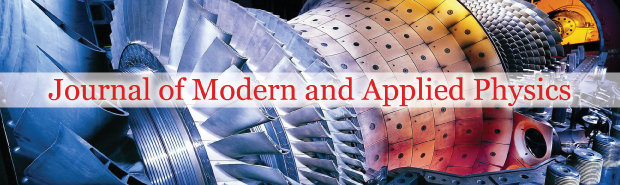All submissions of the EM system will be redirected to Online Manuscript Submission System. Authors are requested to submit articles directly to Online Manuscript Submission System of respective journal.Ashim Saha*

Ashutosh Sastri Road, Kolkata�700010, West Bengal, India, Email: g.saha.ashim@gmail.com

*Correspondence: Ashim Saha, Ashutosh Sastri Road, Kolkata�700010, West Bengal, India, Email: g.saha.ashim@gmail.com

Received: 02-Sep-2022, Manuscript No. PULJMAP-22-5296; Editor assigned: 02-Sep-2022, Pre QC No. PULJMAP-22-5296(PQ); Accepted Date: Sep 13, 2022; Reviewed: 03-Sep-2022 QC No. PULJMAP-22-5296(Q); Revised: 05-Sep-2022, Manuscript No. PULJMAP-22-5296(R); Published: 20-Sep-2022

Citation: Saha A. Understanding of forces between atomic particles by introduction of a new particle type in space. J Mod Appl Phys. 2022; 6(5):1-2.

This open-access article is distributed under the terms of the Creative Commons Attribution Non-Commercial License (CC BY-NC) (http://creativecommons.org/licenses/by-nc/4.0/), which permits reuse, distribution and reproduction of the article, provided that the original work is properly cited and the reuse is restricted to noncommercial purposes. For commercial reuse, contact reprints@pulsus.com

## Abstract

In this paper it has been studied how do forces act between stable atomic particles. To do the findings it has been postulated that a tiny particle type is running throughout the space with some constant velocity. The particle of that type is so tiny that those particles almost don’t collide with each other and hence at any point in space if a solid atomic particle is being kept then the pressure on that atomic particle from all angles are same. However, if a second atomic particle is being brought closer to that former atomic particle, then shadows form on both atomic particles by each other atomic particles. Hence, pressure difference happens, and attraction force is being perceived. Also, it has been postulated that all atomic particles are made of this tiny particle type. As atomic particle spins it scatters those tiny particle and repulsive force is being perceived. In this paper this postulate has been verified mathematically and finally arrived at a formula of net force acting between two stable atomic particles. This mathematical model is successfully predicting change pattern of atomic radius with change in atomic numbers. Also, it is conforming that velocity of light is constant. This same model matches the Lennard-Jones potential function pattern between two atoms.

### Key Words

Positive mass theorem; Negative matter; Repulsion; Dark matter; Dark energy ;Calculation; Inflation; Test.

### Introduction

The following postulates have been considered to find out how does gravitational force work:

• A tiny particle type is running throughout the space with constant velocity.

• The above-mentioned particle type is so tiny that those particles do not collide with each other in free space. That is on any point in space the pressure due to this particle is same from all directions.

Since inside atoms most of the space is empty, and the above-mentioned particles are tiny enough and hence passes though atoms with very little obstruction. That is this tiny particle type passes or flooded though solid object also with very little obstructions. Denser the object is and bigger the object is, more it creates obstruction.

That is - obstruction α volume of object × density of object = mass of object

Hence, though pressure on each object from all direction due to that tiny particle type movement is same, when two solid objects come closer to each other, then an obstruction forms as shadow and hence due to that pressure imbalance gravitational force is being perceived.

Derivation of gravitational force

Let there be two spherical objects, one bigger and one smaller of radius RB and Rs respectively. That is RB>RS . Let those objects volume be VB and Vs respectively. Also let that the respective average densities of those two objects are DB and Ds. It is also assumed that MB and Ms are respective masses of the objects and d is the distance between centers of two spherical objects. Then considering the following figure a mathematical derivation is being made.

Let VR be the volume of the smaller object under shadow. Then VR can be calculated using following integral.The angle α, in above integral is given by following figure,

Now,This d’ is the virtual line of shadow intersecting bigger object. Hence, d'DB is proportional the total shadow effect for all points in smaller object at that angle α. This factor is also required to be multiplied by factor D_S to get total deficiency of pressure in the angle α.

But at each hollow slice cone of these volume ' VR , only cosα factor of the shadow force effect works towards the bigger object. So, the above factor will be multiplied by cos α also. Hence, the net force that small object feels towards big object as follows.Hence net gravitation force acting between these two objects are given by–The above equation 1 looks like Sir Isaac Newton’s formula for gravitational force given below.### Conclusion

Based on the postulates that such tiny particle type exists, it is inferred that this is probably how gravitational force works. However, the source of origin of such tiny particle type is yet to be identified.

###### Journal of Modern and Applied Physics peer review process verified at publonsTop